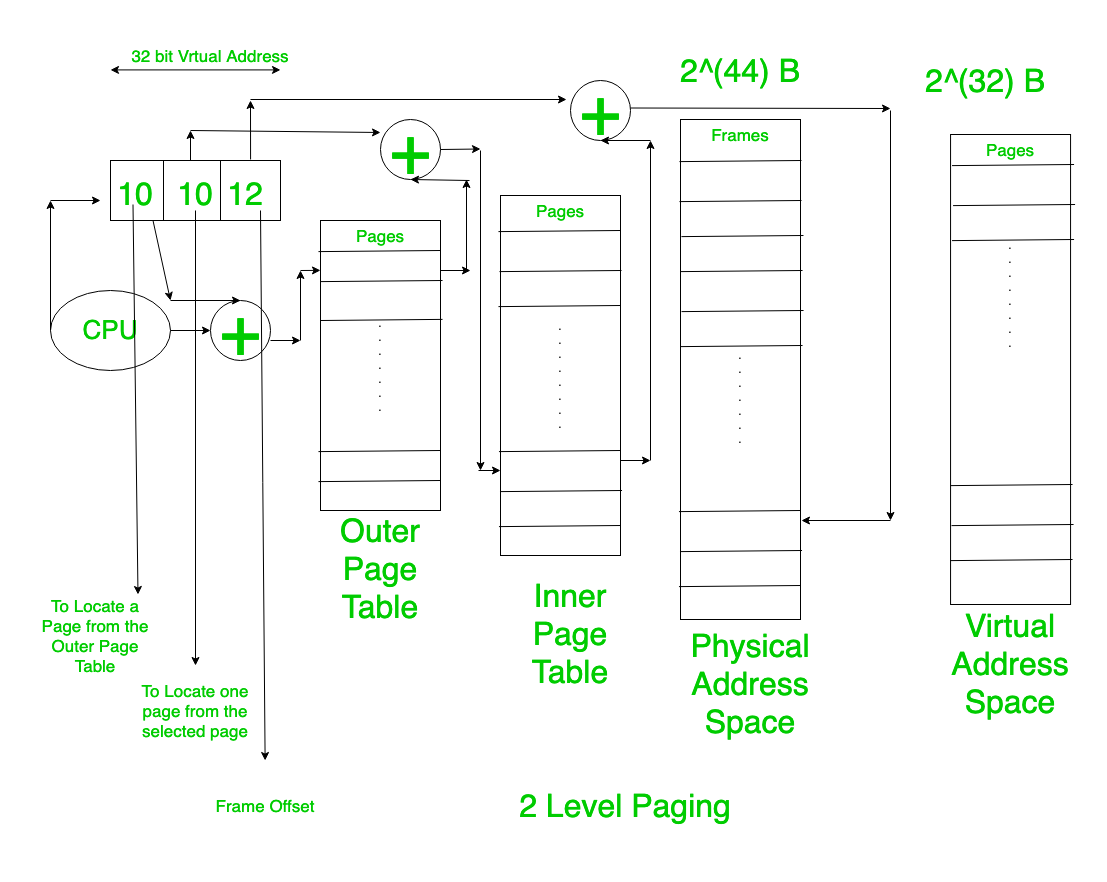# Two Level Paging and Multi Level Paging in OS

Paging is the process in which we convert the entire process in to equal sized pages. Each page further consists of a fixed number of words (if it is word addressable).

The Pages are represented by the Virtual Address generated by the CPU. These Pages are mapped to Physical Address by the MMU. So, to help in this mapping we use the concept of Page Table. Just Like array, in page Table the indices represent the Page Numbers and the contents contain the address of the Frame Number where the the process is actually loaded in the main memory. Because the virtual/Logical Address is relative to zero and should be mapped to actual physical Address Space of Main Memory.

The size of the page table can be calculated from the fact that size of each of entry of page table is given else if it is not given then we can find the number of bits required to address each frame in the main memory (this is not correct but should be used when nothing is given in question).

Page Table Size = No. of Page Table Entries(Total no. of Pages) * (size of each entry of page table)

When the Size of page table is less than the size of one Frame then we need not worry because we can directly put the page table in a frame of the main memory .Thus we can directly access the page table.

But if the size of the page table is larger than the size of Frame. Then the page table in return is to be divided into several pages and these pages of the page table are to be stored in the main memory. Thus, a Outer Page Table comes into the picture.

This Outer Page Table would contain the address of the Frames which contain the pages of Inner Page Table (i.e., Page Table one pages) in the main memory.

The size of this Outer Page is also calculated in the same way as explained above and was used to calculate the size of inner page Table. Now if the size of inner page Table is Less Than or Equal to size of a Frame then we can stop here as we are able to keep the outer most table in a Single frame.

This is called as Two Level Paging.

Example:
Consider Given,

```Physical Address Space = 2(44) B
Virtual Address Space = 2(32) B

Page Entry = 4B
Page Size = 4Kb

So, No.of Frame = 2(32)
No. of Pages Of the Process = 2(20)
Page Table 1 size =2(20) * 4 B= 4 MB ```

As, it is larger than 4B(Frame size).Thus, this Page Table has to converted to pages

No. of pages of the Page Table 2 (Outer Page Table)

`= 2(22)*2(-12)= 2(10) pages `

So, Size of Outer Page Table

`= 2(10) * 4B = 4KB `

Thus here our Outer Page Table (Page Table 2) can be stored in one frame .

Thus, we can stop here.

This is Two-level Paging because here we got 2 page tables.But If still the size of page table is more than the Frame Size then we have to continue till we reach a stage where the size of Outer Most Table is less than the Frame Size. This Concept is called as MultiLevel Paging. Our Target should be to keep the outer most Page Table in a single Frame.

Don’t stop now and take your learning to the next level. Learn all the important concepts of Data Structures and Algorithms with the help of the most trusted course: DSA Self Paced. Become industry ready at a student-friendly price.

My Personal Notes arrow_drop_upCheck out this Author's contributed articles.

If you like GeeksforGeeks and would like to contribute, you can also write an article using contribute.geeksforgeeks.org or mail your article to contribute@geeksforgeeks.org. See your article appearing on the GeeksforGeeks main page and help other Geeks.

Please Improve this article if you find anything incorrect by clicking on the "Improve Article" button below.